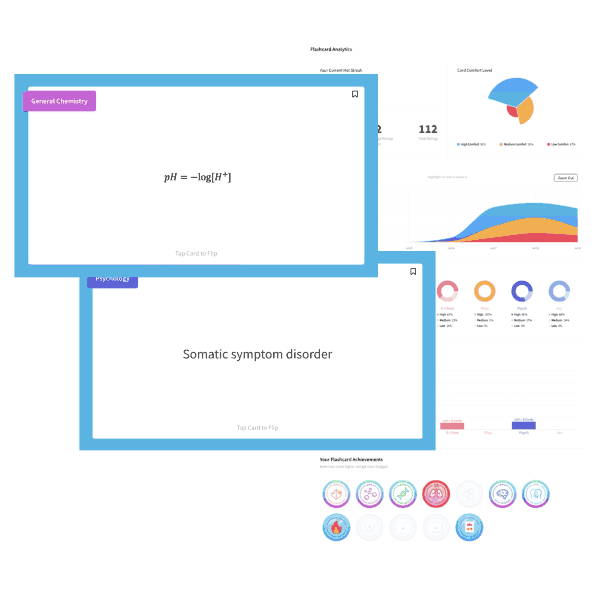# MCAT Chemistry Question — Molecular and Empirical Formulas

One mole of an unknown compound is determined to have a molecular weight of 86 grams per mole. The mass percent of the compound is as follows: 55.81% carbon, 6.98% hydrogen, and 37.21% oxygen. What is the empirical formula and molecular formula, respectively, of the unknown compound.

1. C2H3O and C4H6O2
2. C2H3O and C6H9O3
3. C5H8O and C5H8O
4. C5H8O and C10H16O2
##### Click for Explanation

To answer this question, you must determine the empirical formula of the unknown compound by treating the mass percent as the number of grams of each element out of a 100g sample. The number of moles of each element is determined by the following calculations:

Moles of C = (55.81g C) x (1mol C/12g C) = 4.65 mol C

Moles of H = (6.98g H) x (1 mol H/1g H) = 6.98 mol H

Moles of O = (37.21g O) x (1 mol O/16g O) = 2.33 mol O

Dividing each by the largest common denominator of 2.33 gives the empirical formula of the unknown compound: C2H3O

(4.65/2.33) mol C = 2 mol C

(6.98/2.33) mol H = 3 mol H

(2.33/2.33) mol O = 1 mol O

Since the molecular weight of the compound is 86 g/mol, the correct answer must be A. Note that if you were in a hurry on Test Day, or if you forgot how to solve this problem, you could instead use the periodic table to calculate the molar mass of the molecular formula given in each answer choice. If only one answer gave a molar mass of 86 g/mol (as is the case here, with choice A), then you could be certain that this option was correct. This is true of many MCAT questions: there may be multiple ways to solve the same problem!

## Want more MCAT practice?

### We’ve got options for every schedule and learning style!

From the best online MCAT course created by top instructors with 524+ MCAT scores to the most representative full-length practice exams and private tutoring, we can custom tailor your MCAT prep to your goals!

Not sure which option is right for you? Schedule a free MCAT consultation with an MCAT expert using the form below. No obligation, just expert advice.

Create your Free Account to access our MCAT FlashcardsMCAT is a registered trademark of the Association of American Medical Colleges (AAMC), which is not affiliated with Blueprint.